吉利金鹰静态实拍 6万元的自动挡车型推荐

2014年10月30日 09:18 来源：www.chextx.com 超过：次关注

鹰，是胜利的标志，它象征力量、勇敢、朝气蓬勃。刚刚上市不久的吉利金鹰正是这样的性格，它的力量不是最大的，但是它勇敢，有朝气。金鹰盯住了自动挡家用两厢车这块市场，不过这款车是否会像其名称一样耀眼？下面我们就带您在第一时间近距离接触吉利金鹰。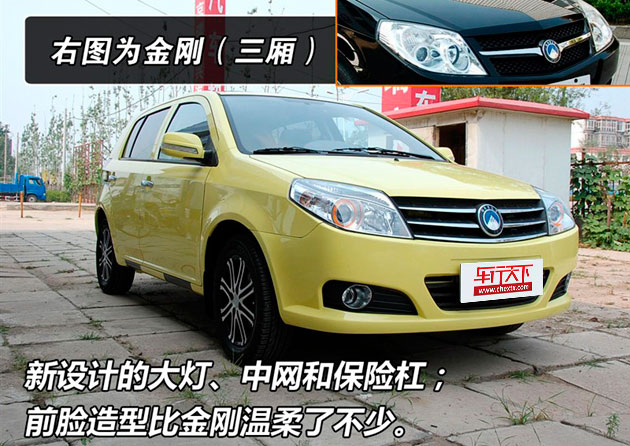第一眼看到金鹰，感觉比想象中的要大一点，而且通过观察可以发现，金鹰不单纯是金刚的两厢版本，很多细节都有改进。比如前脸部分的所有零件都是重新设计的，大灯改为跟宝马一样的带有“眉毛”的天使眼灯，中网改为三条线的镀铬装饰条，保险杠的设计也于三厢的金刚不同。这样的设计使得金鹰的前脸温柔了不少，看起来没有金刚的前脸那么凶，像一只温柔的小雏鹰。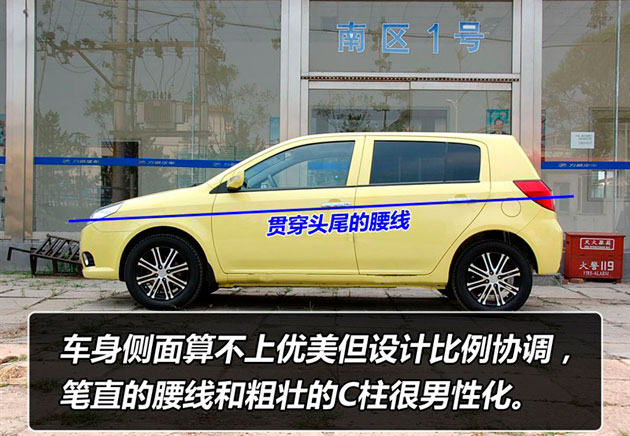金鹰侧面造型平平，没有特别之处，但是设计的干净利落，笔直的腰线从车头一直延伸到车尾，没有弧度，没有断点，这样车身看起来就显得很修长。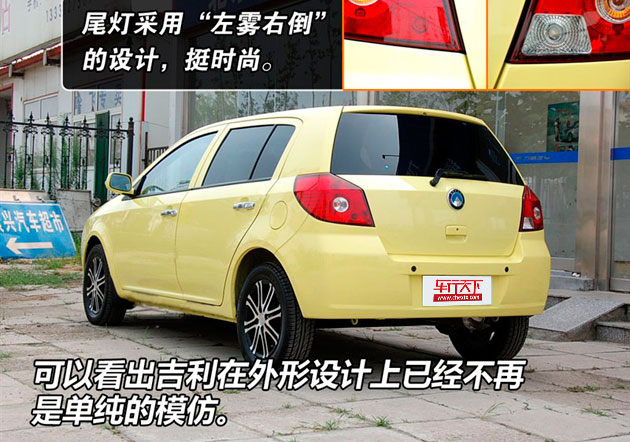既然是新推出的两厢车，那全新的设计就全集中在尾部。金鹰的尾部造型很和谐，没有任何突兀的地方，菱形的尾灯采用了现在比较流行的圆形示宽灯，后雾灯和倒车灯也被分别融入到了左右尾灯里面。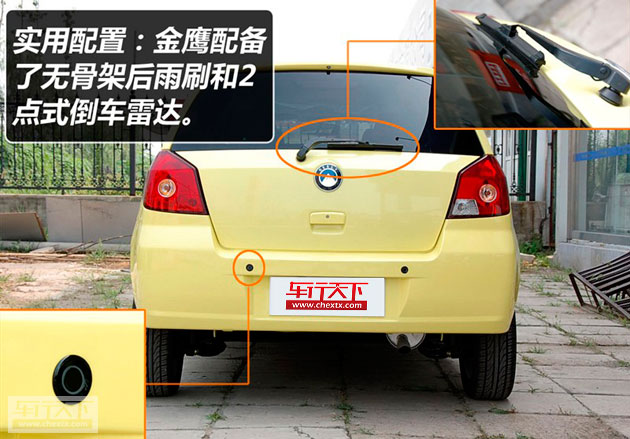后风挡配有无骨架式的雨刷，玻璃上沿还装有玻璃清洗剂喷嘴，这是两厢车必备的，因为两厢车尾部比三厢车会产生更大的空气涡流，在雨天容易把雨水卷到后风挡上，这一点金鹰做得比较周到。倒车雷达虽然为2点式的，但是有总比没有好，好在金鹰的尾部比较短小，视线也不错。金鹰的配置比较齐全，该有的一样没有少。这就是吉利的作风，小型车也要全面，不能因为价格低了就减少配置。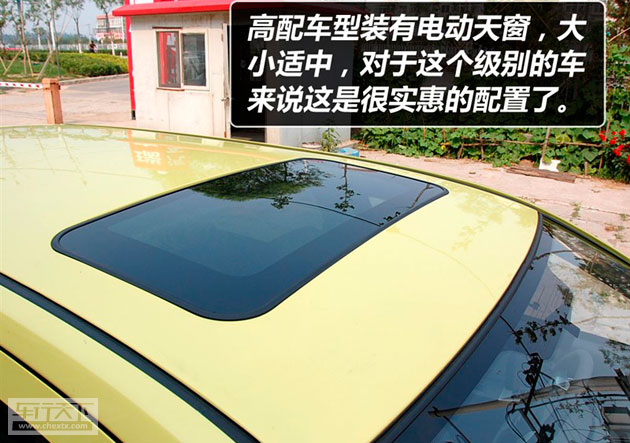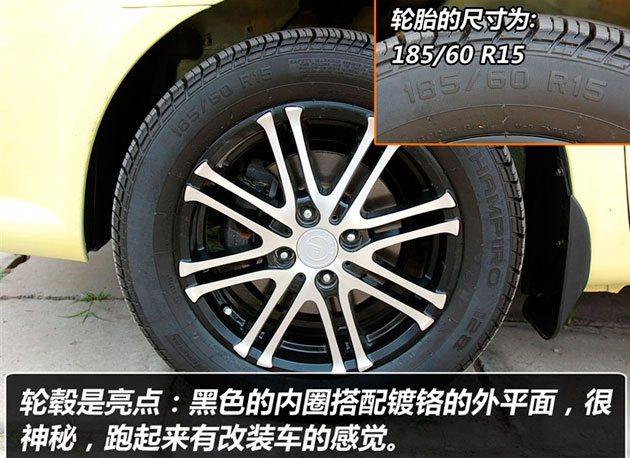从表面上看金鹰，一定会让你有超值的感觉。此车配置了电动天窗，但由于时间原因我们没有做过多的体验，有关天窗的实用感受我们会在以后的文章中提到。金鹰外形上最大的亮点应该说是它的轮毂了。这款轮毂采用的是8辐条中间镂空的设计，造型动感；而喷漆更有特色，轮毂内侧和外圈均为黑色，只有外平面是镀铬的，猛地看上去很像改装车的轮毂，在国产车里这样的设计很少见，使得金鹰独树一帜。

进入车内，会发现金鹰的内饰面板是全新设计的，与金刚轿车的风格完全不同。中控台是横向设计，整体感觉比金刚宽了不少，配上扩张感很强的仿金属银色，显得很大气，很素雅。也有朋友说这款中控台的造型有点像变形金刚，仔细看看还真有那么一点像。还有一处比较明显的改进就是方向盘，金鹰的方向盘为三辐式设计（不知道低配车型是否也是三辐），造型和手感都不错，特别是方向盘上沿的两个小突起，挺有跑车味儿的！辐条上的仿铝质的装饰与内饰的整体风格统一。仪表还是中央设计，很多车友都说当时刚开中置仪表车的时候都很不习惯，但是过了一段时间就觉得跟普通的没什么区别了，而且对于中置仪表来说，眼睛观察仪表的时候是横向的移动，比普通的仪表更适于观察。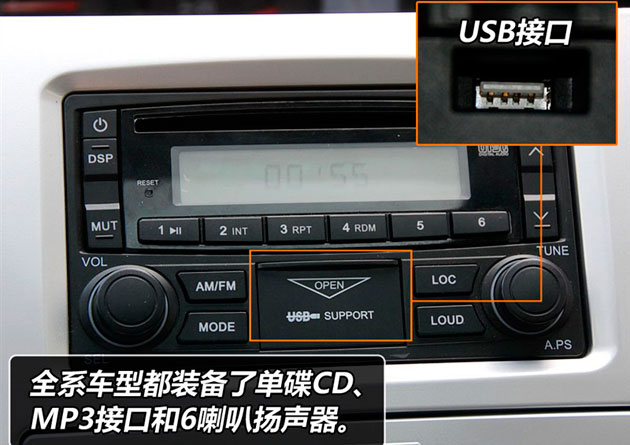音响系统最大的改善就是在机头里整合了USB接口，而且设计的很隐藏。把中控台上的小盖打开后即可把U盘或者MP3播放器插入，需要注意的是，这个插槽很深，完全可以容下一个正常尺寸的U盘，插入后还可以关上盖子，实用且美观；所以如果您的U盘很小的话最好栓一个小绳，这样可以方便的取出。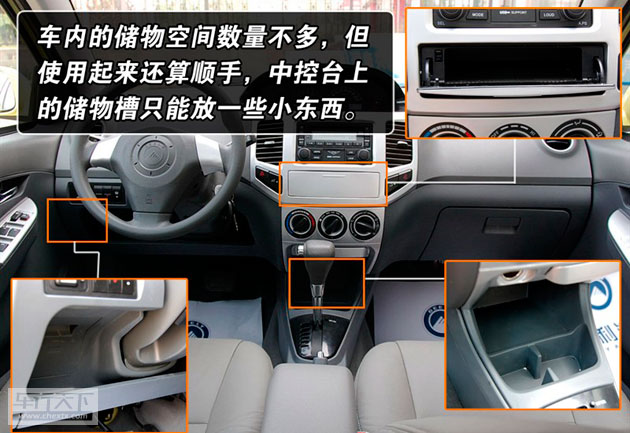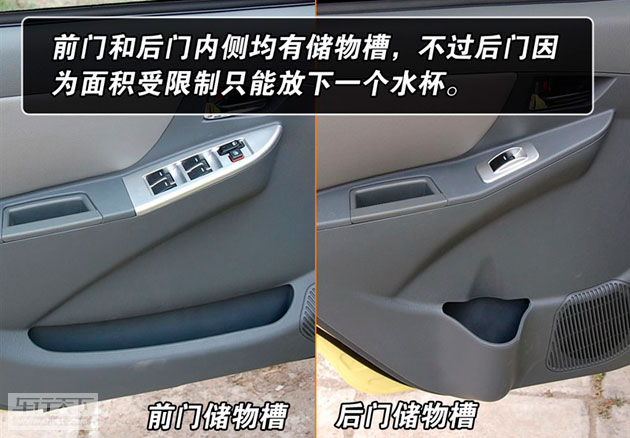金鹰的储物空间不算多，但是用起来还算顺手，除了手套箱以外，在方向盘下、变速箱杆前面和中控台上都有储物槽，不过开口有点小，只能放一些小东西，如果您还有更多的随身物品就放到中央扶手或者门板内侧的储物槽里吧。

自主品牌在短时间内发展到现在的水平是可喜的，吉利金鹰的性价比很高，但如果吉利的装配水平和做工精细程度稍加提高就更好了。也许您还会说金鹰轿车的很多不足之处，但是它价值6.68万元，拥有1.5升发动机、自动变速箱、天窗、真皮座椅……这些还不够吗？

目前来看，在6～8万元这个区间内是最多家庭购车的选择范围，金鹰的出现正是为这些朋友们多带来了一个实惠的选择。

﻿
• 快速找车
• 选择品牌
• 选择品牌
• A  奥迪
• A  阿斯顿·马丁
• A  阿尔法·罗密欧
• B  宝沃
• B  布加迪
• B  巴博斯
• B  保时捷
• B  宾利
• B  奔驰
• B  宝马
• B  本田
• B  别克
• B  标致
• B  比亚迪
• B  宝骏
• B  北汽制造
• B  北汽新能源
• B  北汽幻速
• B  北汽威旺
• B  北京汽车
• B  奔腾
• B  北汽绅宝
• B  北汽昌河
• C  长安欧尚
• C  长安
• C  长安凯程
• C  长城
• D  大众
• D  道奇
• D  DS
• D  东南
• D  东风风神
• D  东风风行
• D  东风小康
• D  东风风度
• D  东风
• F  福特
• F  丰田
• F  菲亚特
• F  法拉利
• F  福田
• F  福迪
• F  福汽启腾
• G  观致
• G  广汽传祺
• G  广汽吉奥
• G  GMC
• H  红旗
• H  汉腾汽车
• H  哈弗
• H  哈飞
• H  海格
• H  海马
• H  华颂
• H  黄海
• H  华泰
• H  恒天
• J  捷途
• J  几何汽车
• J  捷达
• J  吉利汽车
• J  捷豹
• J  Jeep
• J  江淮
• J  江铃
• J  金杯
• J  九龙
• J  金旅
• K  凯翼
• K  凯迪拉克
• K  克莱斯勒
• K  科尼塞克
• K  卡威
• K  开瑞
• L  路虎
• L  林肯
• L  劳斯莱斯
• L  兰博基尼
• L  雷克萨斯
• L  铃木
• L  领克
• L  雷诺
• L  理念
• L  力帆
• L  莲花汽车
• L  猎豹
• L  路特斯
• L  陆风
• M  马自达
• M  MG
• M  MINI
• M  玛莎拉蒂
• M  摩根
• M  迈凯轮
• N  纳智捷
• O  欧拉
• O  欧宝
• O  讴歌
• O  欧朗
• Q  奇瑞
• Q  起亚
• Q  启辰
• R  日产
• R  荣威
• R  瑞麒
• S  SERES赛力斯
• S  三菱
• S  斯威汽车
• S  萨博
• S  smart
• S  斯柯达
• S  斯巴鲁
• S  思铭
• S  双龙
• S  上汽大通
• S  双环
• T  特斯拉
• T  腾势
• W  蔚来
• W  沃尔沃
• W  WEY
• W  五菱汽车
• W  五十铃
• W  威兹曼
• W  威麟
• X  现代
• X  雪佛兰
• X  星途
• X  雪铁龙
• X  小鹏汽车
• X  西雅特
• Y  一汽
• Y  英菲尼迪
• Y  英致
• Y  依维柯
• Y  野马汽车
• Y  永源
• Z  众泰
• Z  中华
• Z  中兴
• Z  知豆
• 选择车系
• 选择车系
• 车型对比
• 选择品牌
• 选择品牌
• A  奥迪
• A  阿斯顿·马丁
• A  阿尔法·罗密欧
• B  宝沃
• B  布加迪
• B  巴博斯
• B  保时捷
• B  宾利
• B  奔驰
• B  宝马
• B  本田
• B  别克
• B  标致
• B  比亚迪
• B  宝骏
• B  北汽制造
• B  北汽新能源
• B  北汽幻速
• B  北汽威旺
• B  北京汽车
• B  奔腾
• B  北汽绅宝
• B  北汽昌河
• C  长安欧尚
• C  长安
• C  长安凯程
• C  长城
• D  大众
• D  道奇
• D  DS
• D  东南
• D  东风风神
• D  东风风行
• D  东风小康
• D  东风风度
• D  东风
• F  福特
• F  丰田
• F  菲亚特
• F  法拉利
• F  福田
• F  福迪
• F  福汽启腾
• G  观致
• G  广汽传祺
• G  广汽吉奥
• G  GMC
• H  红旗
• H  汉腾汽车
• H  哈弗
• H  哈飞
• H  海格
• H  海马
• H  华颂
• H  黄海
• H  华泰
• H  恒天
• J  捷途
• J  几何汽车
• J  捷达
• J  吉利汽车
• J  捷豹
• J  Jeep
• J  江淮
• J  江铃
• J  金杯
• J  九龙
• J  金旅
• K  凯翼
• K  凯迪拉克
• K  克莱斯勒
• K  科尼塞克
• K  卡威
• K  开瑞
• L  路虎
• L  林肯
• L  劳斯莱斯
• L  兰博基尼
• L  雷克萨斯
• L  铃木
• L  领克
• L  雷诺
• L  理念
• L  力帆
• L  莲花汽车
• L  猎豹
• L  路特斯
• L  陆风
• M  马自达
• M  MG
• M  MINI
• M  玛莎拉蒂
• M  摩根
• M  迈凯轮
• N  纳智捷
• O  欧拉
• O  欧宝
• O  讴歌
• O  欧朗
• Q  奇瑞
• Q  起亚
• Q  启辰
• R  日产
• R  荣威
• R  瑞麒
• S  SERES赛力斯
• S  三菱
• S  斯威汽车
• S  萨博
• S  smart
• S  斯柯达
• S  斯巴鲁
• S  思铭
• S  双龙
• S  上汽大通
• S  双环
• T  特斯拉
• T  腾势
• W  蔚来
• W  沃尔沃
• W  WEY
• W  五菱汽车
• W  五十铃
• W  威兹曼
• W  威麟
• X  现代
• X  雪佛兰
• X  星途
• X  雪铁龙
• X  小鹏汽车
• X  西雅特
• Y  一汽
• Y  英菲尼迪
• Y  英致
• Y  依维柯
• Y  野马汽车
• Y  永源
• Z  众泰
• Z  中华
• Z  中兴
• Z  知豆
• 选择车系
• 选择车系
• 选择车型
• 选择车型
• 意见反馈